# Excel Applications for Accounting Principles

## Quiz 7 : Inventory Cost Flow Assumptions FifolifoLooking for Accounting Homework Help?

## Quiz 7 :Inventory Cost Flow Assumptions Fifolifo

Question TypePROBLEM DATA Del Rio began Rio Enterprises on January 1 with 200 units of inventory. During the year, 500 additional units were purchased, 500 units were sold, and Del ended the year with 200 units. Del is very satisfied with his first year of business although the cost of replacing his inventory rose continually throughout the year. The 500 units sold for a total of $320,000 and the 500 units purchased to replace them cost$256,000, so his cash account has increased by $64,000. Del is concerned however because he has three obligations yet to meet: taxes, dividends, and his wife. Federal and state income taxes will take 40% of his income. His investors are to receive dividends equal to half of any income after taxes are paid. And finally, Del promised his wife a big trip to Hawaii if she let him quit his job as a professor and start his own business. He promised her he'd "make at least$50,000 after taxes. That will give us $25,000 after paying off the investors." Del kept fairly good records during the year and knows the specific cost of each inventory unit sold. He has prepared the following table to summarize his purchases and sales.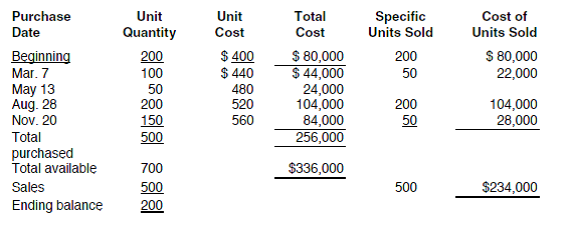A quick calculation shows that Rio's net income will be$51,600 using specific costs for the inventory sold. Sales minus cost of goods sold equals gross profit ($320,000 -$234,000 = $86,000). Taxes to be paid are 40% ($86,000 .4 = $34,400). Subtract taxes from gross profit to get net income ($86,000 - $34,400 =$51,600). Next, Del calculates his ending cash balance. He currently has $64,000 from his sales less his inventory replacement purchases ($320,000 - $256,000 =$64,000). He needs to pay taxes ($34,400) and dividends to his investors ($51,600 .5 = $25,800). Subtracting$34,400 and $25,800 from$64,000 leaves him with only $3,800. Yikes! Del is shocked by the computations. He cannot figure out how he will ever explain to his wife that he has net income in excess of$50,000 and yet after paying off the investors he will have only $3,800 to show for it! Del knows you are taking an accounting class and comes to you for help. REQUIREMENT Del has heard that the choice of an inventory cost flow assumption can have a significant effect on net income and taxes. He asks you to show him the differences between the specific identification method and the cost flow assumptions of FIFO, LIFO, and weighted average methods. Review the worksheet FIFOLIFO that follows these requirements. Note that all of the problem data have been entered in the Data Section of the worksheet. Free Essay Answer: Answer: In this problem, cost of goods sold and ending inventory is to be determined using various different methods. The required file worksheet is shown below::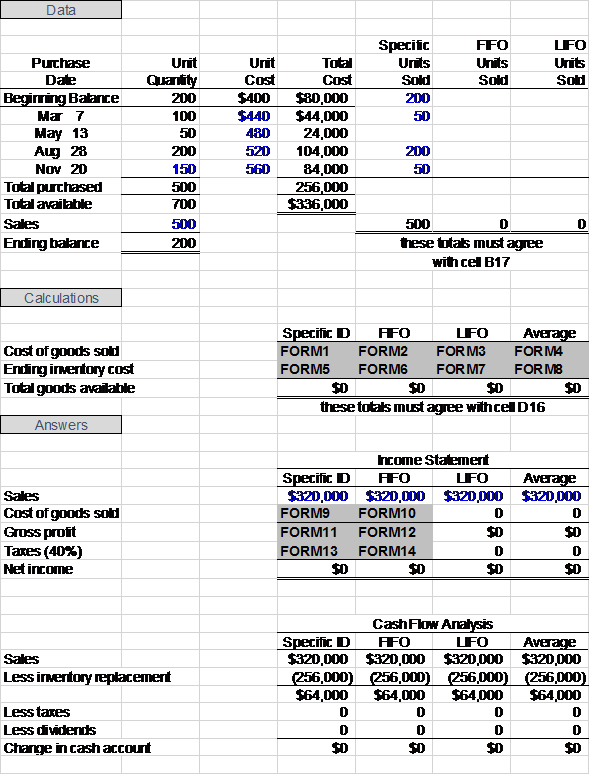TagsPROBLEM DATA Del Rio began Rio Enterprises on January 1 with 200 units of inventory. During the year, 500 additional units were purchased, 500 units were sold, and Del ended the year with 200 units. Del is very satisfied with his first year of business although the cost of replacing his inventory rose continually throughout the year. The 500 units sold for a total of$320,000 and the 500 units purchased to replace them cost $256,000, so his cash account has increased by$64,000. Del is concerned however because he has three obligations yet to meet: taxes, dividends, and his wife. Federal and state income taxes will take 40% of his income. His investors are to receive dividends equal to half of any income after taxes are paid. And finally, Del promised his wife a big trip to Hawaii if she let him quit his job as a professor and start his own business. He promised her he'd "make at least $50,000 after taxes. That will give us$25,000 after paying off the investors." Del kept fairly good records during the year and knows the specific cost of each inventory unit sold. He has prepared the following table to summarize his purchases and sales.A quick calculation shows that Rio's net income will be $51,600 using specific costs for the inventory sold. Sales minus cost of goods sold equals gross profit ($320,000 - $234,000 =$86,000). Taxes to be paid are 40% ($86,000 .4 =$34,400). Subtract taxes from gross profit to get net income ($86,000 -$34,400 = $51,600). Next, Del calculates his ending cash balance. He currently has$64,000 from his sales less his inventory replacement purchases ($320,000 -$256,000 = $64,000). He needs to pay taxes ($34,400) and dividends to his investors ($51,600 .5 =$25,800). Subtracting $34,400 and$25,800 from $64,000 leaves him with only$3,800. Yikes! Del is shocked by the computations. He cannot figure out how he will ever explain to his wife that he has net income in excess of $50,000 and yet after paying off the investors he will have only$3,800 to show for it! Del knows you are taking an accounting class and comes to you for help. REQUIREMENT Using a pencil, fill in columns F and G in the Data Section of the worksheet printout at the end of this problem.
Free
Essay

In the open worksheet file, record the inventory sold using FIFO and LIFO in the appropriate columns. In FIFO (First In First Out) method, the first units of inventory purchased are the first units to be taken out of inventory. In LIFO (Last In First Out) method, the most recent units purchased will be the first units to be taken out of inventory.
The required table is shown below: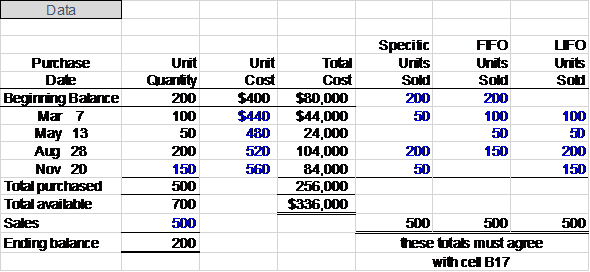TagsPROBLEM DATA Del Rio began Rio Enterprises on January 1 with 200 units of inventory. During the year, 500 additional units were purchased, 500 units were sold, and Del ended the year with 200 units. Del is very satisfied with his first year of business although the cost of replacing his inventory rose continually throughout the year. The 500 units sold for a total of $320,000 and the 500 units purchased to replace them cost$256,000, so his cash account has increased by $64,000. Del is concerned however because he has three obligations yet to meet: taxes, dividends, and his wife. Federal and state income taxes will take 40% of his income. His investors are to receive dividends equal to half of any income after taxes are paid. And finally, Del promised his wife a big trip to Hawaii if she let him quit his job as a professor and start his own business. He promised her he'd "make at least$50,000 after taxes. That will give us $25,000 after paying off the investors." Del kept fairly good records during the year and knows the specific cost of each inventory unit sold. He has prepared the following table to summarize his purchases and sales.A quick calculation shows that Rio's net income will be$51,600 using specific costs for the inventory sold. Sales minus cost of goods sold equals gross profit ($320,000 -$234,000 = $86,000). Taxes to be paid are 40% ($86,000 .4 = $34,400). Subtract taxes from gross profit to get net income ($86,000 - $34,400 =$51,600). Next, Del calculates his ending cash balance. He currently has $64,000 from his sales less his inventory replacement purchases ($320,000 - $256,000 =$64,000). He needs to pay taxes ($34,400) and dividends to his investors ($51,600 .5 = $25,800). Subtracting$34,400 and $25,800 from$64,000 leaves him with only $3,800. Yikes! Del is shocked by the computations. He cannot figure out how he will ever explain to his wife that he has net income in excess of$50,000 and yet after paying off the investors he will have only $3,800 to show for it! Del knows you are taking an accounting class and comes to you for help. REQUIREMENT Open the file FIFOLIFO from the website for this book at cengagebrain.com. Fill in columns F and G in the Data Section. Then enter all 14 formulas where indicated on the worksheet. Be careful to write FORMULAS 1 through 8 broadly enough to include different sales quantities (i.e., suppose all inventory was sold or no inventory was sold). Enter your name in cell A1. Save the results as FIFOLIFO3. Print the worksheet when done. Also print your formulas. Check figure: FIFO net income (cell E36),$56,400.
Free
Essay

In the calculations portion of the worksheet, formulas will be used to calculate the cost of goods sold and ending inventory cost using the specific identification, FIFO, LIFO, and average weight methods.
For specific identification method, the actual cost of the units sold will be used as per the information in the data section. For the FIFO method, the unit costs from the first units purchased in inventory will be used. For the LIFO method, the unit costs from the last units purchased in inventory will be used. For the average weighted method, average of costs of all inventory purchases will be used as a per-unit cost. The required worksheet is shown below: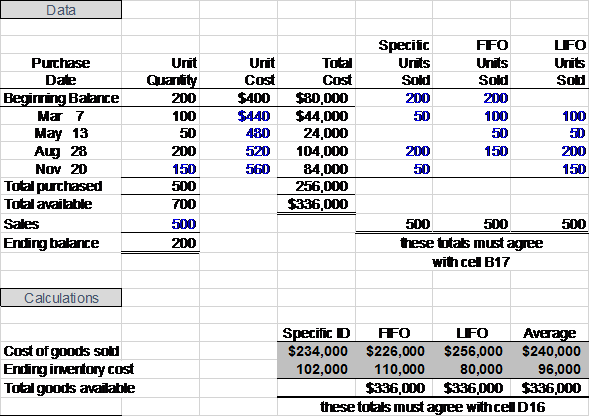Total goods available will have same value in for all the methods.
In the answers section of the worksheet, formulas will be used to calculate costs of goods sold, gross profit, and taxes. Cost of goods sold is already calculated, cell reference will be used for those formulas. To calculate gross profit, cost of goods sold will be subtracted from sales. To calculate taxes, gross profit will be multiplied by 40%. Cell references will be used in the formulas so that they will change automatically if a cell value changes. The required worksheet is given below: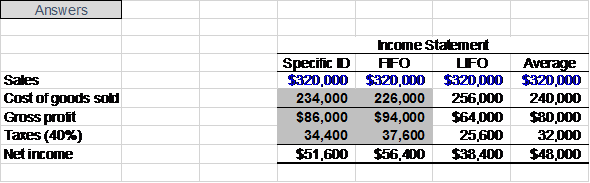Different methods of inventory accounting will result in different net incomes.

TagsPROBLEM DATA Del Rio began Rio Enterprises on January 1 with 200 units of inventory. During the year, 500 additional units were purchased, 500 units were sold, and Del ended the year with 200 units. Del is very satisfied with his first year of business although the cost of replacing his inventory rose continually throughout the year. The 500 units sold for a total of $320,000 and the 500 units purchased to replace them cost$256,000, so his cash account has increased by $64,000. Del is concerned however because he has three obligations yet to meet: taxes, dividends, and his wife. Federal and state income taxes will take 40% of his income. His investors are to receive dividends equal to half of any income after taxes are paid. And finally, Del promised his wife a big trip to Hawaii if she let him quit his job as a professor and start his own business. He promised her he'd "make at least$50,000 after taxes. That will give us $25,000 after paying off the investors." Del kept fairly good records during the year and knows the specific cost of each inventory unit sold. He has prepared the following table to summarize his purchases and sales.A quick calculation shows that Rio's net income will be$51,600 using specific costs for the inventory sold. Sales minus cost of goods sold equals gross profit ($320,000 -$234,000 = $86,000). Taxes to be paid are 40% ($86,000 .4 = $34,400). Subtract taxes from gross profit to get net income ($86,000 - $34,400 =$51,600). Next, Del calculates his ending cash balance. He currently has $64,000 from his sales less his inventory replacement purchases ($320,000 - $256,000 =$64,000). He needs to pay taxes ($34,400) and dividends to his investors ($51,600 .5 = $25,800). Subtracting$34,400 and $25,800 from$64,000 leaves him with only $3,800. Yikes! Del is shocked by the computations. He cannot figure out how he will ever explain to his wife that he has net income in excess of$50,000 and yet after paying off the investors he will have only $3,800 to show for it! Del knows you are taking an accounting class and comes to you for help. REQUIREMENT Examine your completed worksheet and answer the following questions: a. Which inventory cost flow assumption produces the most net income b. Which inventory cost flow assumption produces the least net income c. What caused the difference between your answers to a and b d. Which inventory cost flow assumption produces the highest ending cash balance e. Which inventory cost flow assumption produces the lowest ending cash balance f. Does the assumption that produces the highest net income also produce the highest cash balance Explain. g. As you recall, Del originally used the specific identification method in his initial calculations when he projected$51,600 net income. According to Del's reckoning, that should have left him cash of $25,800 (50% of$51,600) after paying his investors. Why would he only have $3,800 left Explain. h. Which inventory cost flow assumption would you suggest Del use Explain. Essay Answer: TagsWHAT-IF ANALYSIS What changes would have taken place if Del's purchase prices had fallen rather than risen To find out, enter the following values in cells C11 through C14, respectively: 390, 380, 370, and 360. Print the results. Explain what the changes are and why they have taken place. Essay Answer: TagsWHAT-IF ANALYSIS Suppose Del's purchase prices had remained constant. Enter 400 in cells C11 through C14. Explain what changes take place and why. Essay Answer: TagsWHAT-IF ANALYSIS Reset the purchase prices to their original values (cells C11 through C14). Suppose Del had purchased 250 units on November 20 rather than 150. Enter 250 in cell C14 and alter column G in the Data Section. Explain what happens to net income under each inventory cost flow assumption and why. Also, what "management" implications might this have for Del Essay Answer: TagsWHAT-IF ANALYSIS Reset the November 20 purchase to 150 units, including column G. To test your formulas, suppose that Del had sold 600 units rather than 500. Sales now total$384,000. The extra units sold come from the May 13 purchase (25 units) and the November 20 purchase (75 units). Change cell B17 to 600 and cells D32 through G32 to \$384,000. Alter columns E, F, and G in the Data Section to reflect the change. Your formulas should automatically redo the Calculations and Answer sections. Print the results again.
Essay